# Setup

library(ggtreeExtra)
library(ggstar)
library(ggplot2)
library(ggtree)
library(treeio)
library(ggnewscale)

# 1. Introduction

The geom_facet function provided in the ggtree package (Yu et al. 2017) was designed to align associated graphs to the tree (Yu et al. 2018). It only works with rectangular, roundrect, ellipse and slanted layouts to present tree data on external panels. To extend ggtree to present associated data on the outer rings of phylogenetic tree in circular, fan and radial layouts, we developed ggtreeExtra, which is able to align associated graphs with a tree in rectangular, circular, fan and radial layouts.

The ggtreeExtra package provides a function, geom_fruit, to align graphs to a tree. The associated graphs will align at different positions of the external panels of the tree. We also developed geom_fruit_list to add multiple layers at the same external panel of tree. Furthermore, axis of an external layer can be added using the axis.params=list(axis="x",...) in geom_fruit. Background grid lines of an external layer can be added using the grid.params=list() in geom_fruit. These functions are based on ggplot2 and support using grammar of graphics (Wickham 2016). See also Chapter10 of the online book.

# 2. Install

You can use the following commands to install it

# for devel
if(!requireNamespace("remotes", quietly=TRUE)){
install.packages("remotes")
}
remotes::install_github("YuLab-SMU/ggtreeExtra")

# for release
if (!requireNamespace("BiocManager", quietly=TRUE))
install.packages("BiocManager")

## BiocManager::install("BiocUpgrade") ## you may need this
BiocManager::install("ggtreeExtra")

# 3. Usage

To demonstrate the usages of the package, we use a tree file downloaded from plotTree with simulated associated datasets. All the simulated datasets contain one column of taxa labels of the tree.

# The path of tree file.
trfile <- system.file("extdata", "tree.nwk", package="ggtreeExtra")
# The path of file to plot tip point.
tippoint1 <- system.file("extdata", "tree_tippoint_bar.csv", package="ggtreeExtra")
# The path of first layer outside of tree.
ring1 <- system.file("extdata", "first_ring_discrete.csv", package="ggtreeExtra")
# The path of second layer outside of tree.
ring2 <- system.file("extdata", "second_ring_continuous.csv", package="ggtreeExtra")

# The tree file was imported using read.tree. If you have other tree format files, you can use corresponding functions from treeio package to read it.

# This dataset will to be plotted point and bar.
knitr::kable(head(dat1))
ID Location Length Group Abundance
DE0655_HCMC_2001 HK 0.1786629 Yes 12.331055
MS0111_HCMC_1996 HK 0.2105236 Yes 9.652052
MS0063_HCMC_1995 HK 1.4337983 Yes 11.584822
DE0490_HCMC_2000 HK 0.3823731 Yes 7.893231
DE0885_HCMC_2001 HK 0.8478901 Yes 12.117232
DE0891_HCMC_2001 HK 1.5038646 Yes 10.819734
# This dataset will to be plotted heatmap
knitr::kable(head(dat2))
ID Pos Type
DE0846_HCMC_2001 8 type2
MS0034_HCMC_1995 8 type2
EG1017_HCMC_2009 6 type2
KH18_HCMC_2009 5 type2
10365_HCMC_2010 7 type2
EG1021_HCMC_2009 1 type1
# This dataset will to be plotted heatmap
knitr::kable(head(dat3))
ID Type2 Alpha
MS0004_HCMC_1995 p3 0.2256195
DE1150_HCMC_2002 p2 0.2222086
MS0048_HCMC_1995 p2 0.1881510
HUE57_HCMC_2010 p3 0.1939088
DE1486_HCMC_2002 p2 0.2018493
DE1165_HCMC_2002 p3 0.1812997
# The format of the datasets is the long shape for ggplot2. If you have short shape dataset,
# you can use melt of reshape2 or pivot_longer of tidyr to convert it.

# We use ggtree to create fan layout tree.
p <- ggtree(tree, layout="fan", open.angle=10, size=0.5)
#> Scale for y is already present.
#> Adding another scale for y, which will replace the existing scale.
p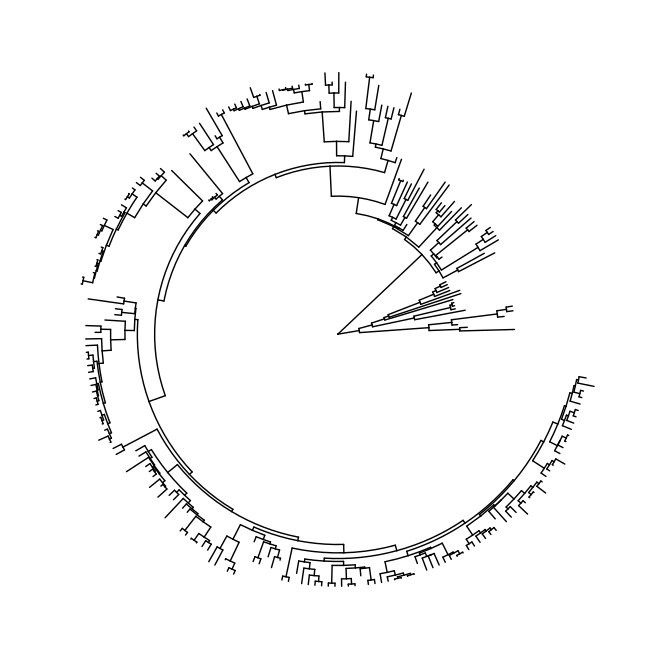## Next, we can use %<+% of ggtree to add annotation dataset to tree.
#p1 <- p %<+% dat1
#p1
## We use geom_star to add point layer outside of tree.
#p2 <- p1 +
#      geom_star(
#          mapping=aes(fill=Location, size=Length, starshape=Group),
#          starstroke=0.2
#      ) +
#      scale_size_continuous(
#          range=c(1, 3),
#          guide=guide_legend(
#                     keywidth=0.5,
#                     keyheight=0.5,
#                     override.aes=list(starshape=15),
#                     order=2)
#      ) +
#      scale_fill_manual(
#          values=c("#F8766D", "#C49A00", "#53B400", "#00C094", "#00B6EB", "#A58AFF", "#FB61D7"),
#          guide="none" # don't show the legend.
#      ) +
#      scale_starshape_manual(
#          values=c(1, 15),
#          guide=guide_legend(
#                    keywidth=0.5, # adjust width of legend
#                    keyheight=0.5, # adjust height of legend
#                    order=1 # adjust the order of legend for multiple legends.
#                ),
#          na.translate=FALSE # to remove the NA legend.
#      )
#p2

# Or if you don't use %<+% to import annotation dataset, instead of data parameter of geom_fruit.

# You should specify the column contained tip labels to y axis of mapping, here is y=ID.

# the position parameter was set to identity to add the points on the tip nodes.
p2 <- p +
geom_fruit(
data=dat1,
geom=geom_star,
mapping=aes(y=ID, fill=Location, size=Length, starshape=Group),
position="identity",
starstroke=0.2
) +
scale_size_continuous(
range=c(1, 3), # the range of size.
guide=guide_legend(
keywidth=0.5,
keyheight=0.5,
override.aes=list(starshape=15),
order=2
)
) +
scale_fill_manual(
values=c("#F8766D", "#C49A00", "#53B400", "#00C094", "#00B6EB", "#A58AFF", "#FB61D7"),
guide="none"
) +
scale_starshape_manual(
values=c(1, 15),
guide=guide_legend(
keywidth=0.5,
keyheight=0.5,
order=1
)
)
p2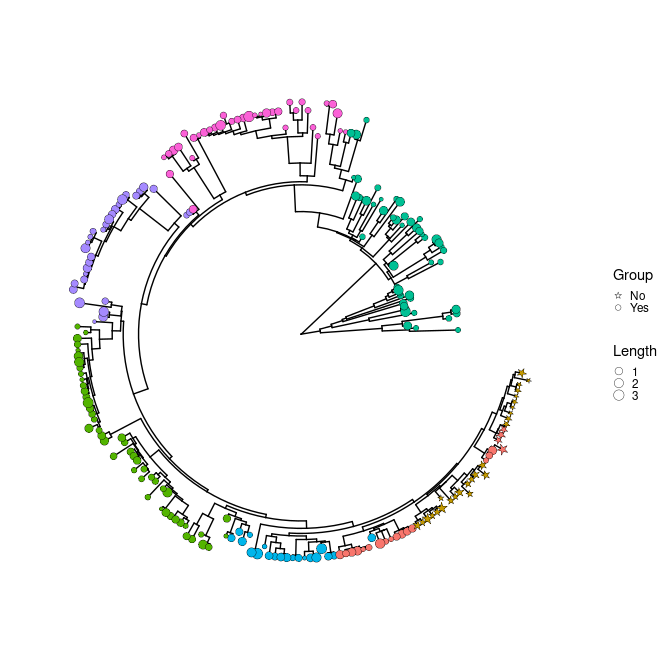# Next, we will add a heatmap layer on the outer ring of p2 using geom_tile of ggplot2.
# Since we want to map some variable of dataset to the fill aesthetics of geom_tile, but
# the fill of p2 had been mapped. So I need use new_scale_fill of ggnewscale package to initialize it.
p3 <- p2 +
new_scale_fill() +
geom_fruit(
data=dat2,
geom=geom_tile,
mapping=aes(y=ID, x=Pos, fill=Type),
offset=0.08,   # The distance between external layers, default is 0.03 times of x range of tree.
pwidth=0.25 # width of the external layer, default is 0.2 times of x range of tree.
) +
scale_fill_manual(
values=c("#339933", "#dfac03"),
guide=guide_legend(keywidth=0.5, keyheight=0.5, order=3)
)
p3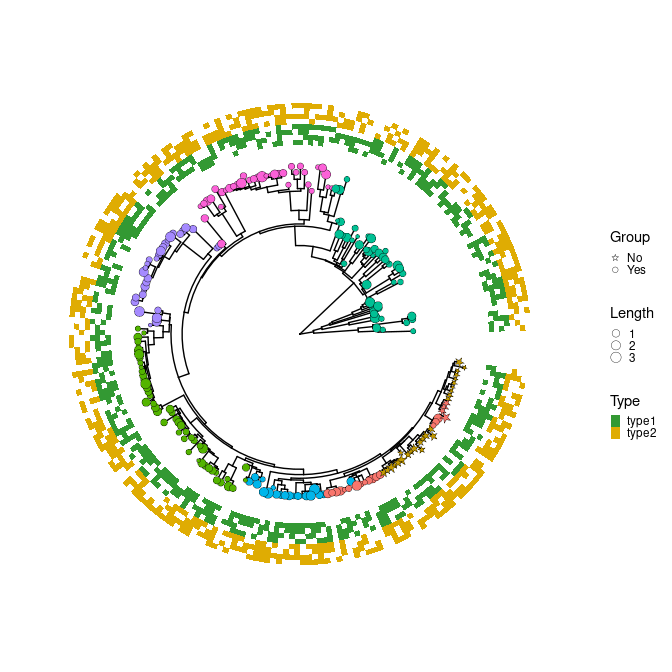# You can also add heatmap layer for continuous values.
p4 <- p3 +
new_scale_fill() +
geom_fruit(
data=dat3,
geom=geom_tile,
mapping=aes(y=ID, x=Type2, alpha=Alpha, fill=Type2),
pwidth=0.15,
axis.params=list(
axis="x", # add axis text of the layer.
text.angle=-45, # the text angle of x-axis.
hjust=0  # adjust the horizontal position of text of axis.
)
) +
scale_fill_manual(
values=c("#b22222", "#005500", "#0000be", "#9f1f9f"),
guide=guide_legend(keywidth=0.5, keyheight=0.5, order=4)
) +
scale_alpha_continuous(
range=c(0, 0.4), # the range of alpha
guide=guide_legend(keywidth=0.5, keyheight=0.5, order=5)
)

# Then add a bar layer outside of the tree.
p5 <- p4 +
new_scale_fill() +
geom_fruit(
data=dat1,
geom=geom_col,
mapping=aes(y=ID, x=Abundance, fill=Location),  #The 'Abundance' of 'dat1' will be mapped to x
pwidth=0.4,
axis.params=list(
axis="x", # add axis text of the layer.
text.angle=-45, # the text size of axis.
hjust=0  # adjust the horizontal position of text of axis.
),
grid.params=list() # add the grid line of the external bar plot.
) +
scale_fill_manual(
values=c("#F8766D", "#C49A00", "#53B400", "#00C094", "#00B6EB", "#A58AFF", "#FB61D7"),
guide=guide_legend(keywidth=0.5, keyheight=0.5, order=6)
) +
theme(#legend.position=c(0.96, 0.5), # the position of legend.
legend.background=element_rect(fill=NA), # the background of legend.
legend.title=element_text(size=7), # the title size of legend.
legend.text=element_text(size=6), # the text size of legend.
legend.spacing.y = unit(0.02, "cm")  # the distance of legends (y orientation).
)
p5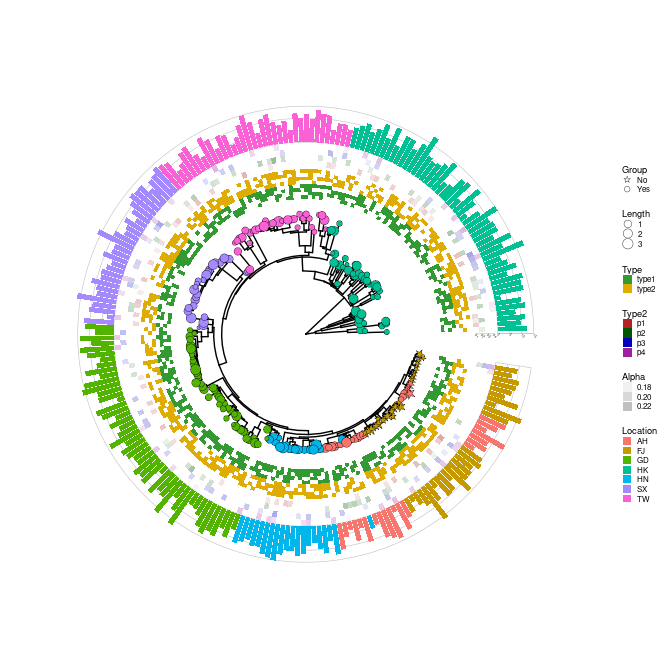## 3.2 add multiple layers on the same position.

In the section, we simulated a tree with several associated datasets, and used geom_fruit_list to add multiple layers at the same external panel of the tree.

# To reproduce.
set.seed(1024)
# generate a tree contained 100 tip labels.
tr <- rtree(100)
# generate three datasets, which are the same except the third column name.
dt <- data.frame(id=tr\$tip.label, value=abs(rnorm(100)), group=c(rep("A",50),rep("B",50)))
df <- dt
dtf <- dt
colnames(df)[] <- "group2"
colnames(dtf)[] <- "group3"
# plot tree
p <- ggtree(tr, layout="fan", open.angle=0)
#> Scale for y is already present.
#> Adding another scale for y, which will replace the existing scale.
p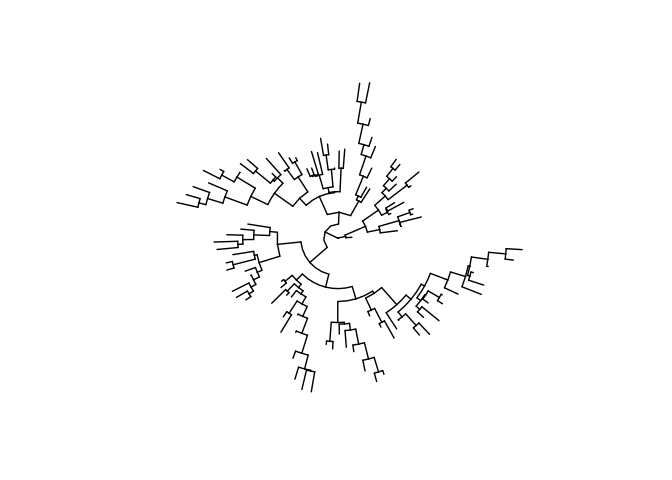# the first ring.
p1 <- p +
geom_fruit(
data = dt,
geom = geom_col,
mapping = aes(y=id, x=value, fill=group),
) +
new_scale_fill()
p1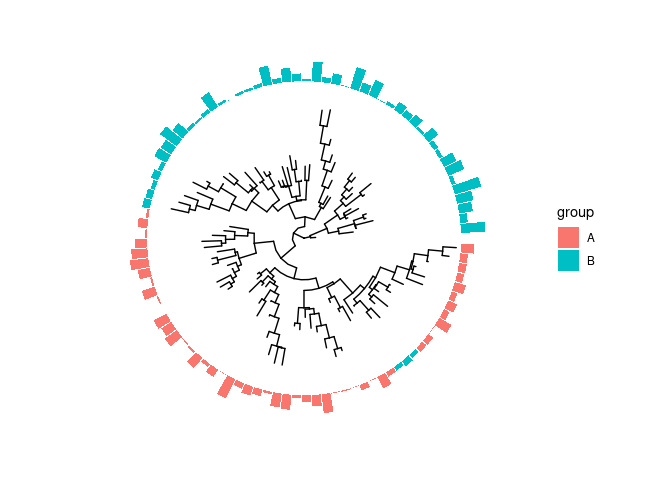# the second ring
# geom_fruit_list is a list, which first element must be layer of geom_fruit.
p2 <- p1 +
geom_fruit_list(
geom_fruit(
data = df,
geom = geom_col,
mapping = aes(y=id, x=value, fill=group2),
),
scale_fill_manual(values=c("blue", "red")), # To map group2
new_scale_fill(), # To initialize fill scale.
geom_fruit(
data = dt,
geom = geom_star,
mapping = aes(y=id, x=value, fill=group),
size = 2.5,
color = NA,
starstroke = 0
)
) +
new_scale_fill()
p2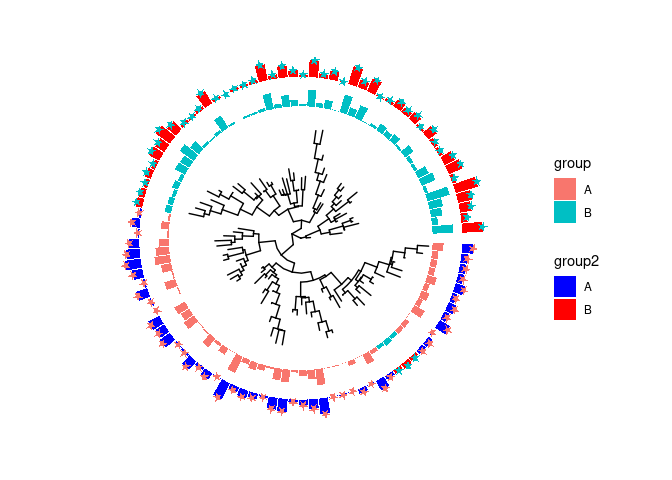# The third ring
p3 <- p2 +
geom_fruit(
data = dtf,
geom = geom_col,
mapping = aes(y=id, x=value, fill=group3),
stat = "identity"
) +
scale_fill_manual(values=c("#00AED7", "#009E73"))
#> Warning in geom_col(data = structure(list(label = c("t1", "t10", "t100", :
#> Ignoring unknown parameters: stat
p3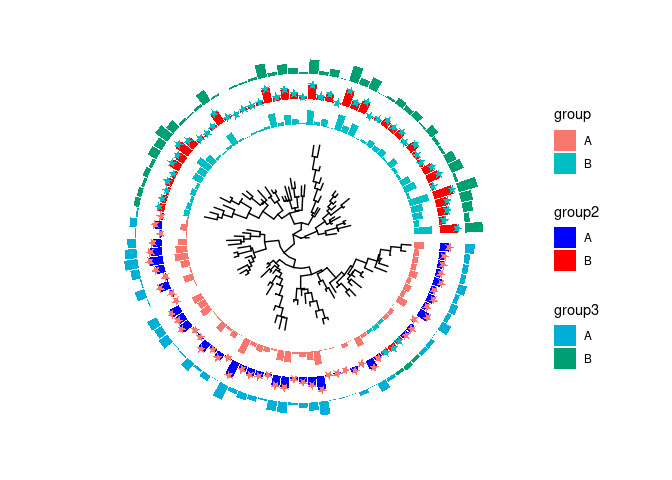# 4. Need helps?

If you have any questions/issues, please visit github issue tracker. You also can post to google group. Users are highly recommended to subscribe to the mailing list.

# 5. Session information

Here is the output of sessionInfo() on the system on which this document was compiled:

#> R version 4.3.1 (2023-06-16)
#> Platform: x86_64-pc-linux-gnu (64-bit)
#> Running under: Ubuntu 22.04.3 LTS
#>
#> Matrix products: default
#> BLAS:   /home/biocbuild/bbs-3.18-bioc/R/lib/libRblas.so
#> LAPACK: /usr/lib/x86_64-linux-gnu/lapack/liblapack.so.3.10.0
#>
#> locale:
#>   LC_CTYPE=en_US.UTF-8       LC_NUMERIC=C
#>   LC_TIME=en_GB              LC_COLLATE=C
#>   LC_MONETARY=en_US.UTF-8    LC_MESSAGES=en_US.UTF-8
#>   LC_PAPER=en_US.UTF-8       LC_NAME=C
#>  LC_MEASUREMENT=en_US.UTF-8 LC_IDENTIFICATION=C
#>
#> time zone: America/New_York
#> tzcode source: system (glibc)
#>
#> attached base packages:
#>  stats     graphics  grDevices utils     datasets  methods   base
#>
#> other attached packages:
#>  ggnewscale_0.4.9   treeio_1.26.0      ggtree_3.10.0      ggplot2_3.4.4
#>  ggstar_1.0.4       ggtreeExtra_1.12.0
#>
#> loaded via a namespace (and not attached):
#>   yulab.utils_0.1.0  sass_0.4.7         utf8_1.2.4         generics_0.1.3
#>   tidyr_1.3.0        prettydoc_0.4.1    ggplotify_0.1.2    lattice_0.22-5
#>   digest_0.6.33      magrittr_2.0.3     evaluate_0.22      grid_4.3.1
#>  fastmap_1.1.1      jsonlite_1.8.7     ape_5.7-1          gridExtra_2.3
#>  purrr_1.0.2        fansi_1.0.5        aplot_0.2.2        scales_1.2.1
#>  lazyeval_0.2.2     jquerylib_0.1.4    cli_3.6.1          rlang_1.1.1
#>  munsell_0.5.0      tidytree_0.4.5     withr_2.5.1        cachem_1.0.8
#>  yaml_2.3.7         tools_4.3.1        parallel_4.3.1     memoise_2.0.1
#>  dplyr_1.1.3        colorspace_2.1-0   vctrs_0.6.4        R6_2.5.1
#>  gridGraphics_0.5-1 lifecycle_1.0.3    fs_1.6.3           ggfun_0.1.3
#>  pkgconfig_2.0.3    pillar_1.9.0       bslib_0.5.1        gtable_0.3.4
#>  glue_1.6.2         Rcpp_1.0.11        xfun_0.40          tibble_3.2.1
#>  tidyselect_1.2.0   knitr_1.44         farver_2.1.1       htmltools_0.5.6.1
#>  nlme_3.1-163       patchwork_1.1.3    labeling_0.4.3     rmarkdown_2.25
#>  compiler_4.3.1

# 6. Reference

Wickham, Hadley. 2016. Ggplot2: Elegant Graphics for Data Analysis. Springer-Verlag New York. https://ggplot2.tidyverse.org.

Yu, Guangchuang, Tommy Tsan-Yuk Lam, Huachen Zhu, and Yi Guan. 2018. “Two Methods for Mapping and Visualizing Associated Data on Phylogeny Using Ggtree.” Molecular Biology and Evolution 35 (2): 3041–3. https://doi.org/10.1093/molbev/msy194.

Yu, Guangchuang, David Smith, Huachen Zhu, Yi Guan, and Tommy Tsan-Yuk Lam. 2017. “Ggtree: An R Package for Visualization and Annotation of Phylogenetic Trees with Their Covariates and Other Associated Data.” Methods in Ecology and Evolution 8 (1): 28–36. https://doi.org/10.1111/2041-210X.12628.